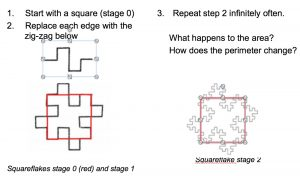1. If the red square has area 1 square unit. What is the area enclosed by the stage 1 squareflake?

2. What is the area enclosed by the stage 2 squareflake? What about the stages 3 and 4 squareflakes?

3. If replacing each line segment on the edge with the zig-zag is repeated infinitely often what is the area enclosed by the fractal squareflake?

4. What is the perimeter of the stage 0 squareflake?

5. The length of each line segment is exactly one quarter of the length of the line segment replaced by the zig-zag. What is the perimeter of the stage 1 squareflake?

6. What is the perimeter of the stage 2 squareflake?

7. What is the perimeter of the stage 3 squareflake?

8. What do you notice about the number pattern for the perimeter as the process is repeated?

9. If replacing each line segment on the edge with the zig-zag is repeated n times what is the perimeter of the stage n squareflake?

10. If replacing each line segment on the edge with the zig-zag is repeated infinitely often what is the area enclosed by the fractal squareflake?